# Making many synthetic seismic models in Python

In this short post I show how to adapt Agile Scientific‘s Python tutorial x lines of code, Wedge model and adapt it to make 100 synthetic models in one shot: X  impedance models times X wavelets times X random noise fields (with I vertical fault).

N.B. the code is optimized for Python 2.7.

I begin by making a 6-layer model, shown in Figure 1:

```model = numpy.zeros((50,49), dtype=np.int)
model[8:16,:] = 1
model[16:24,:] = 2
model[24:32,:] = 3
model[32:40,:] = 4
model[40:,:] = 5```Figure 1. Initial 6-layer model

next I make some Vp-rho pairs (rock 0, rock 1, … , rock5):

```rocks = numpy.array([[2700, 2750],  # Vp, rho
[2400, 2450],
[2600, 2650],
[2400, 2450],
[2800, 3000],
[3100, 3200],])```

and then create 10 slightly different variations of the Vp-rho pairs one of which are is shown in Figure 2:

```rnd = numpy.random.rand(10,6,2)*0.2
manyrocks = np.array([rocks + rocks*rn for rn in rnd], dtype=np.int)
earth = manyrocks[model]```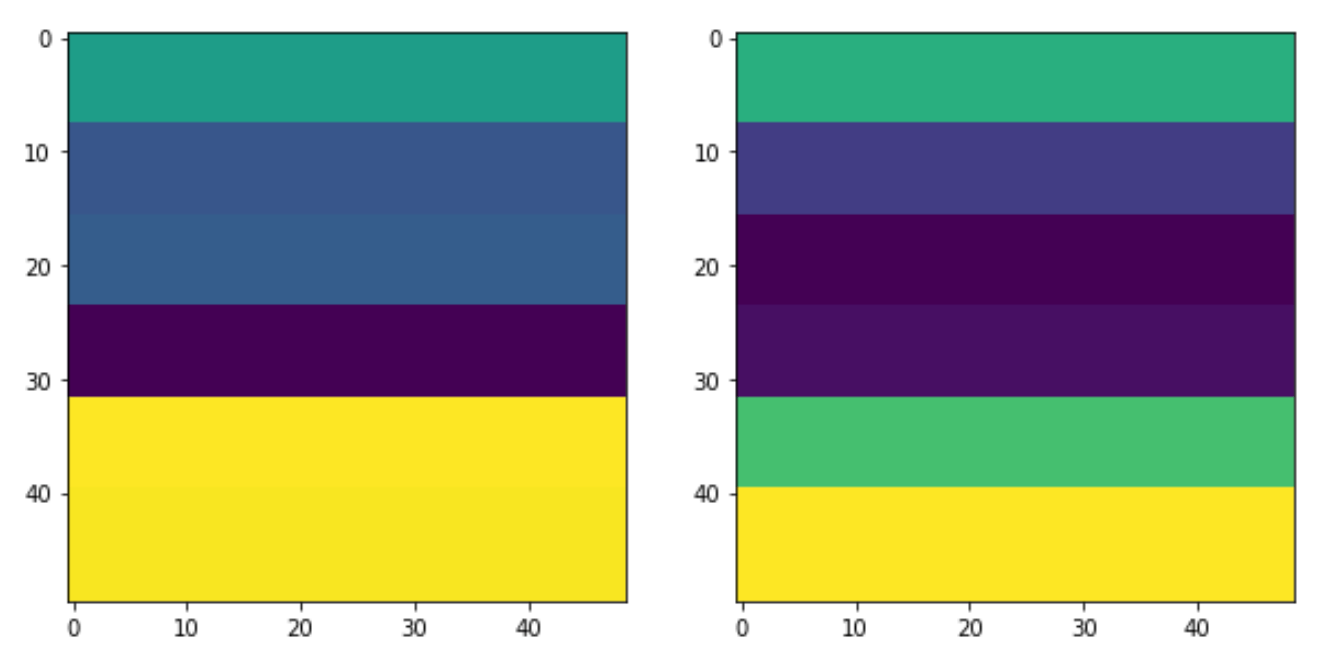Figure 2. A Vp-rho pair (earth model)

at which point I can combine Vp-rho pairs to make 10 impedance models, then insert a vertical fault with:

```impedances = [np.apply_along_axis(np.product, -1, e).astype(float) for e in earth]# Python 2
faulted = copy.deepcopy(impedances)
for r, i in zip(faulted, np.arange(len(faulted))):
temp = np.array(r)
rolled = np.roll(np.array(r[:,:24]), 4, axis = 0)
temp[:,:24]=rolled
faulted[i]=temp```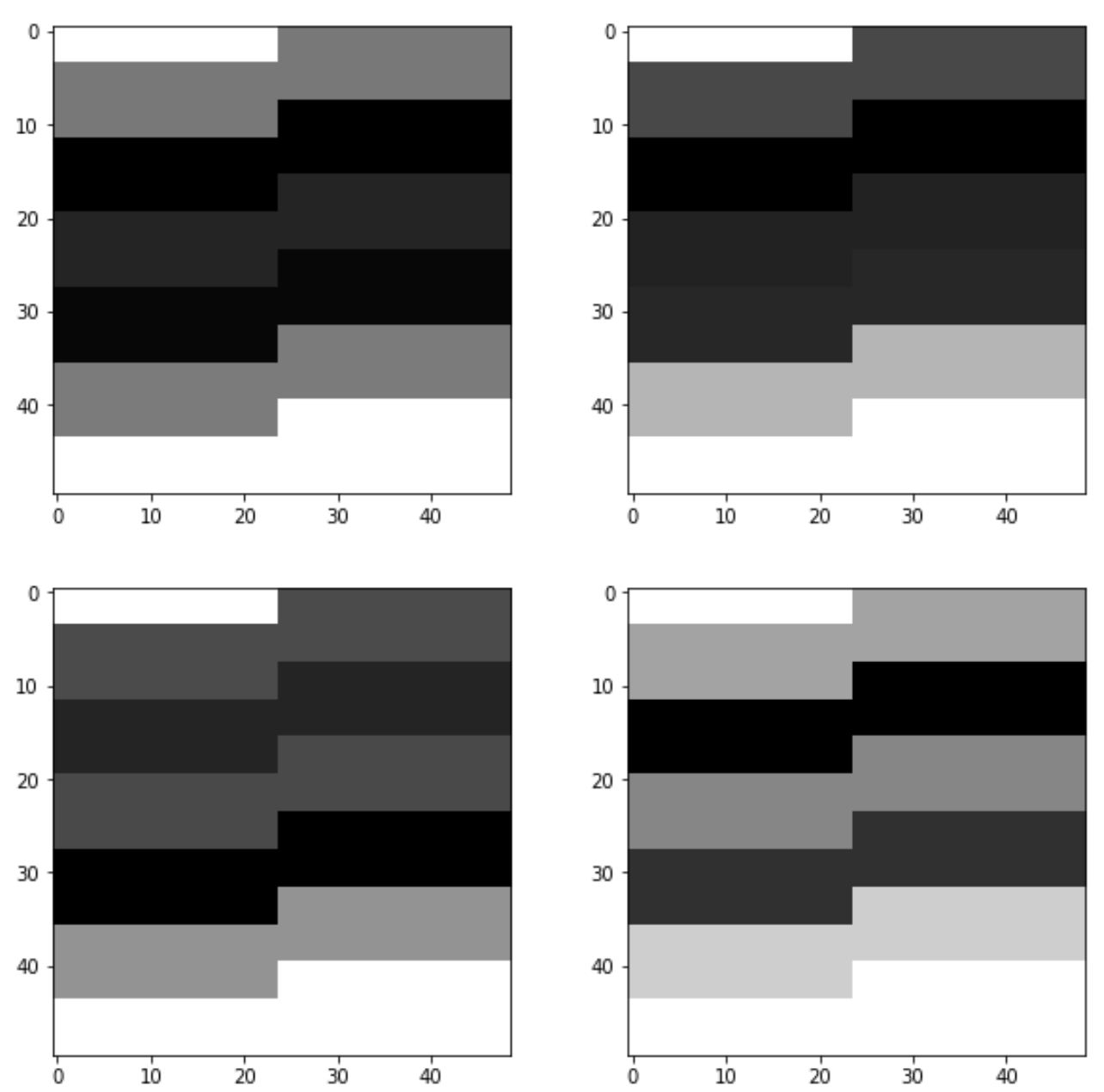Figure 3. Four faulted impedance models.

next I calculate reflection coefficients (Figure 4)and convolve them with a list of 10 Ricker wavelets (generated using Agile’s Bruges) to make synthetic seismic models, shown in Figure 5.

```rc =  [(flt[1:,:] - flt[:-1,:]) / (flt[1:,:] + flt[:-1,:]) for flt in faulted]
ws = [bruges.filters.ricker(duration=0.098, dt=0.002, f=fr)
for fr in [35, 40, 45, 50, 55, 60, 65, 70, 75, 80]]
synth = np.array([np.apply_along_axis(lambda t: np.convolve(t, w, mode='same'), axis=0,
arr=r) for r in rc for w in ws ])
```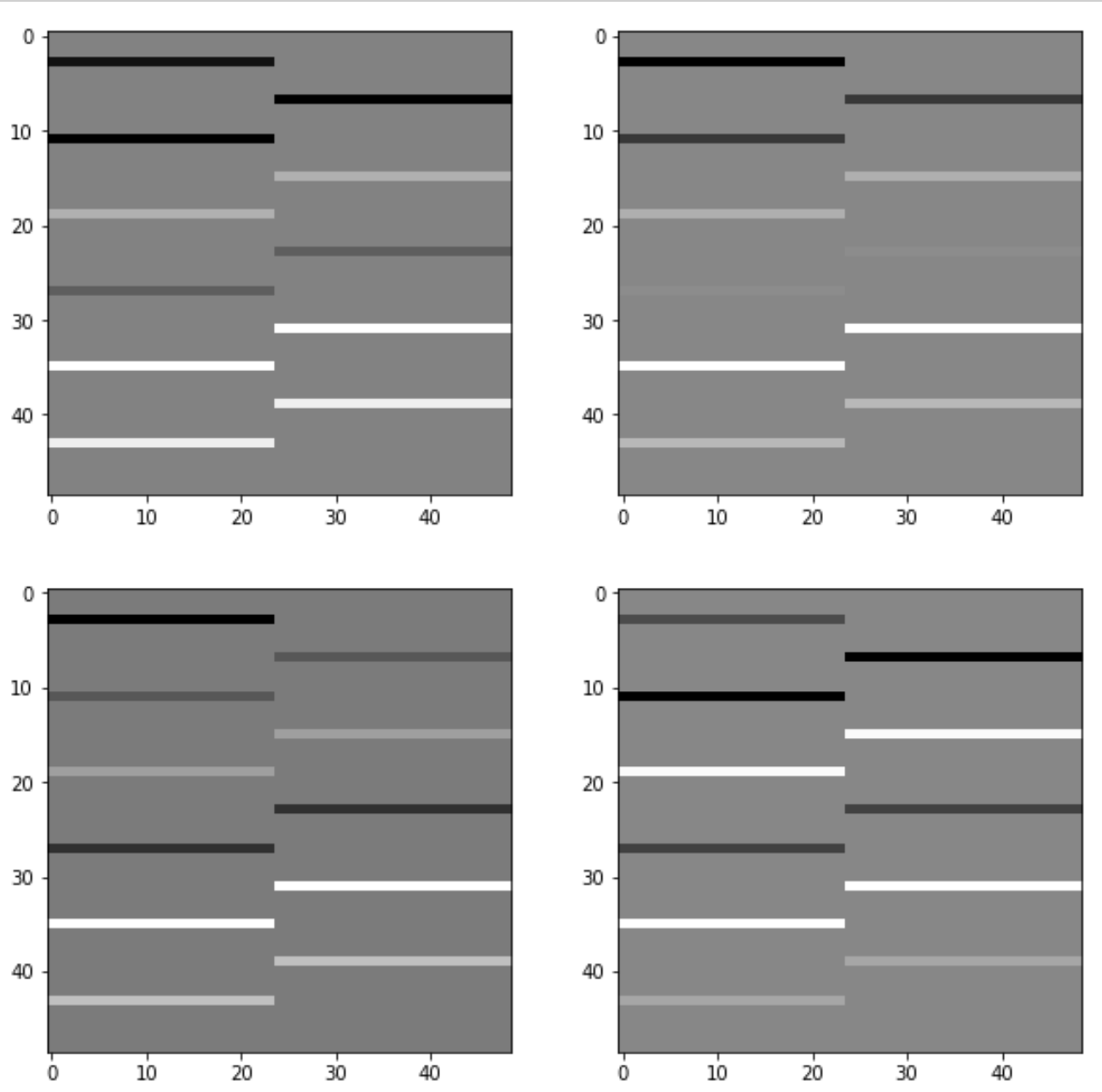Figure 4. Four reflection coefficients series.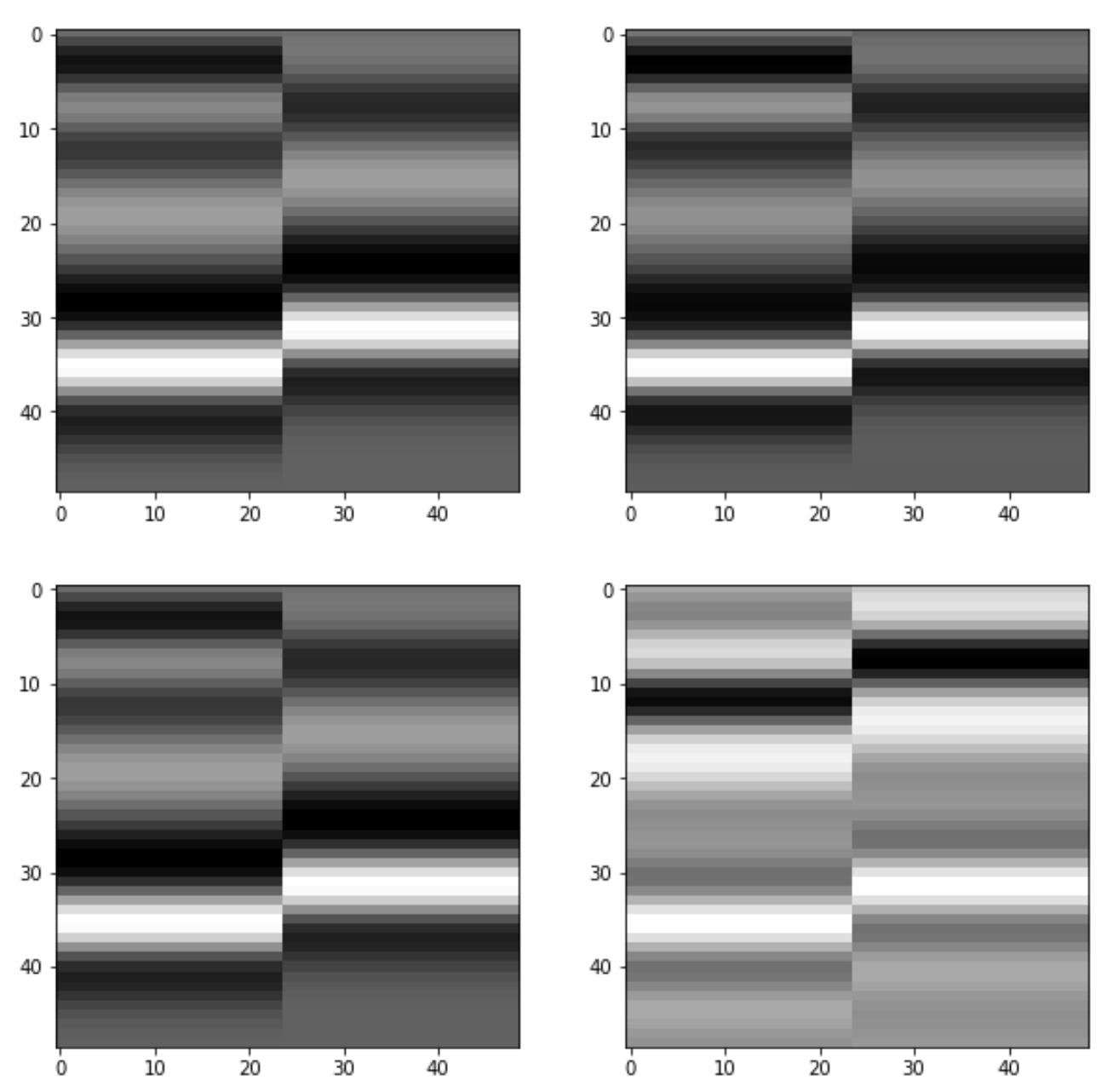Figure 5. Four synthetic seismic models with vertical fault.

The last bit is the addition of noise, with the result is shown in Figure 6:

```blurred = sp.ndimage.gaussian_filter(synth, sigma=1.1)
noisy = blurred + 0.5 * blurred.std() * np.random.random(blurred.shape)```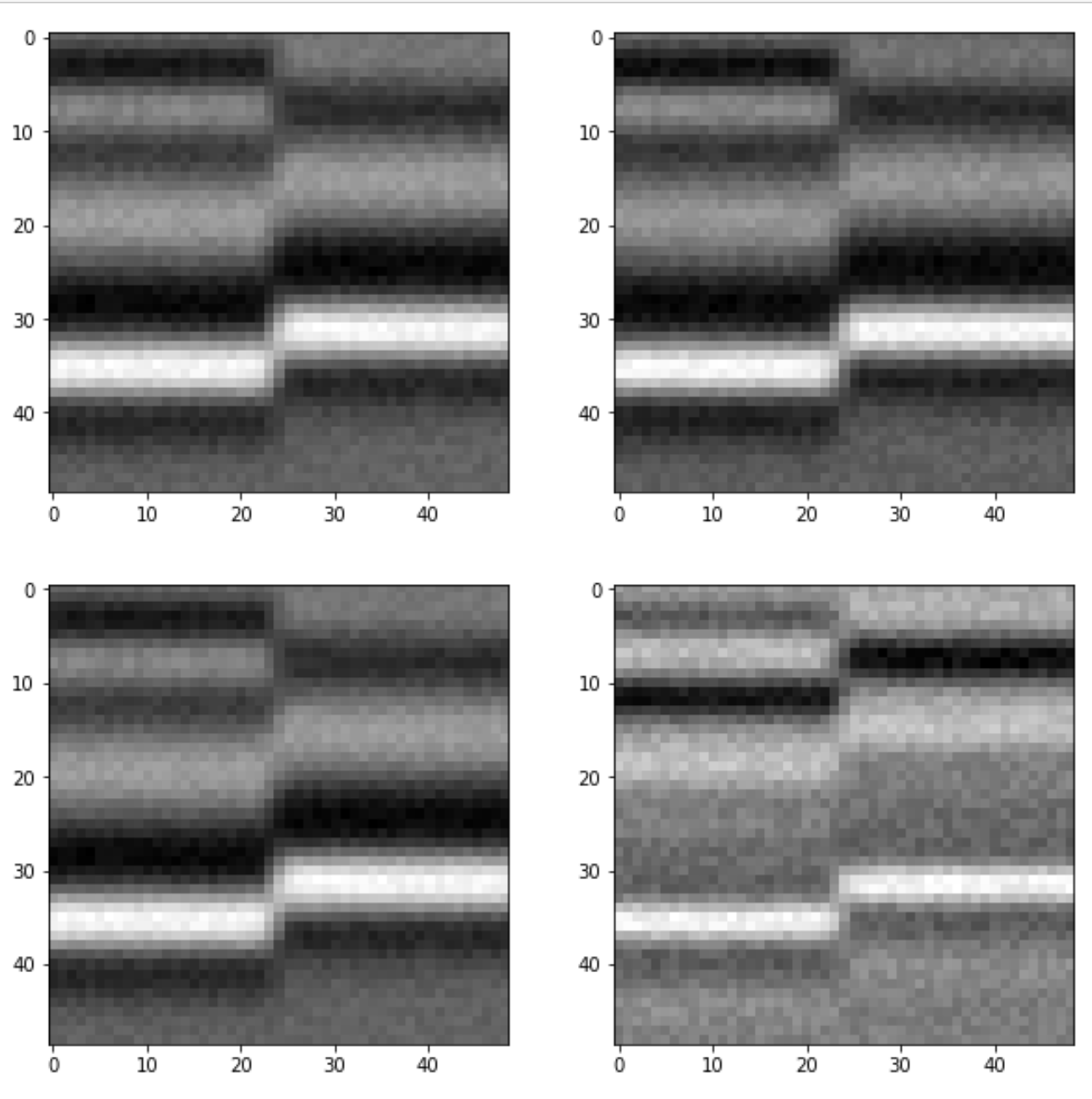Figure 6. Four synthetic seismic models with vertical fault and noise.

Done!

The notebook with the full code is on GitHub, let me know if you find this useful or if you come up with more modeling ideas.

UPDATE, April 22, 2019.

I think the key to understand this is how we multiply velocity by density in each of the possible earth model.

Looking at the notebook, the earth model array has shape:
```print (np.shape(earth))
>>> (10, 50, 49, 2)```
with the last axis having dimension 2: one Vp and one Rho, so in summary 10 models of size 50×49, each with a Vp and a Rho.
So with this other block of code:
```impedances = [np.apply_along_axis(np.product,
-1, e).astype(float) for e in earth]```

you use `numpy.apply_along_axis`  to multiply Vp by Rho along the last dimension, `-1` , using `numpy.product`, and the list comprehension `[... for e in earth]` to do it for all models in the array `earth`.

## 13 thoughts on “Making many synthetic seismic models in Python”

1. hello, i want to ask about the fault on the figure.
1. is there a code to make to fault not vertical?
2. is there a code to count the slip of the fault?
thank you

2.Flavio on said:

Hi Matteo,
very useful notebook, I was looking for something similar, but I’m still struggling to save the models to bin files, I tried to do this:
earth = np.asarray(earth, order=’C’)
for w in range(1,11):
filename = “figures/model{}.bin”.format(w)
with open(filename, ‘wb’) as outfile:
outfile.write(earth)

It seems to save the earth models, but when I use Seismic Unix to open the bin files, that should have as first dimension =50, it give me only a black figure with zeros. Is it wrong what I’m doing?

• Hi Flavio
Unfortunately I’ve never worked with that format, or Seismic Unix for what matters.for that matter. But I can almost guarantee if you ask in the Software Underground Slack group someone will come up with something…
https://softwareunderground.org/slack

•Flavio on said:

Thanks Matteo,

3.Kirill on said:

Dear Matteo,
Thank you for tutorial! I wonder is there any way to generate synthetic seismic image with channel or point bar?
Thank you.

4.DipnStrike on said:

Is there a code to create quality low frequency models ?

• @DipnStrike: I am not sure what you mean by “quality low frequency”

5.Setyo Ari on said:

Hello, do you have a coding for sesimic reflection anisotrophy ?